Ex 8.1

Chapter 8 Class 10 Introduction to Trignometry (Term 1)
Serial order wise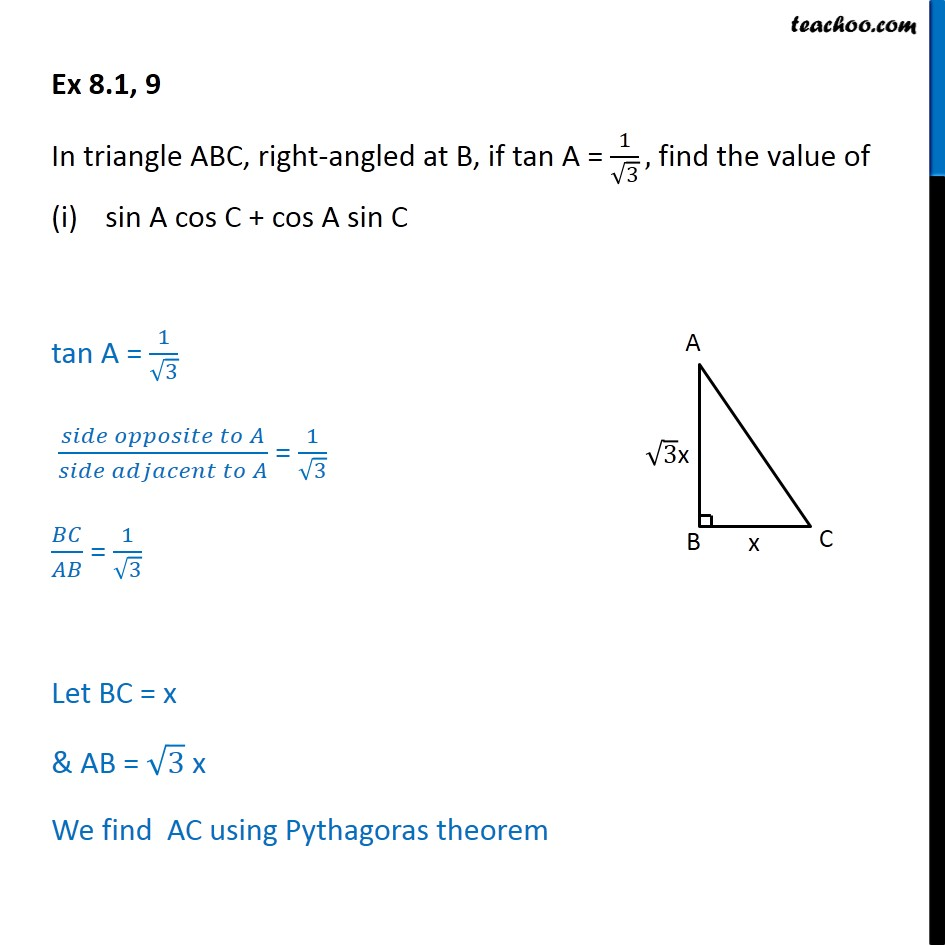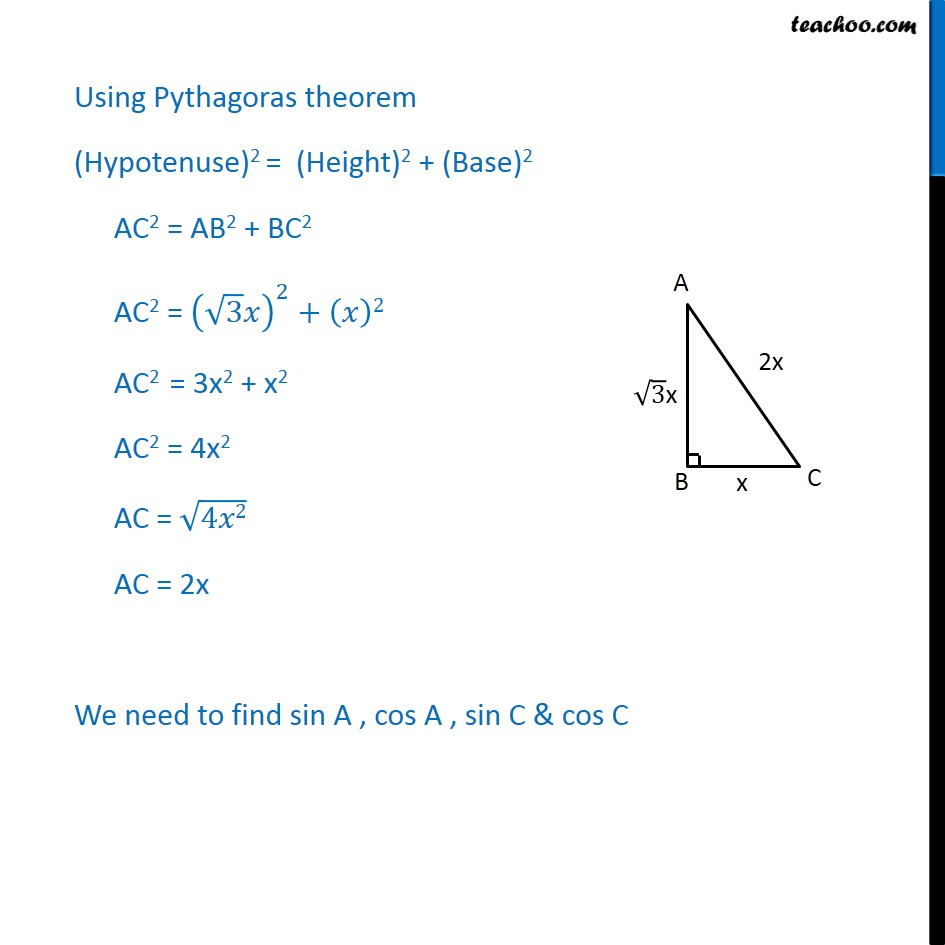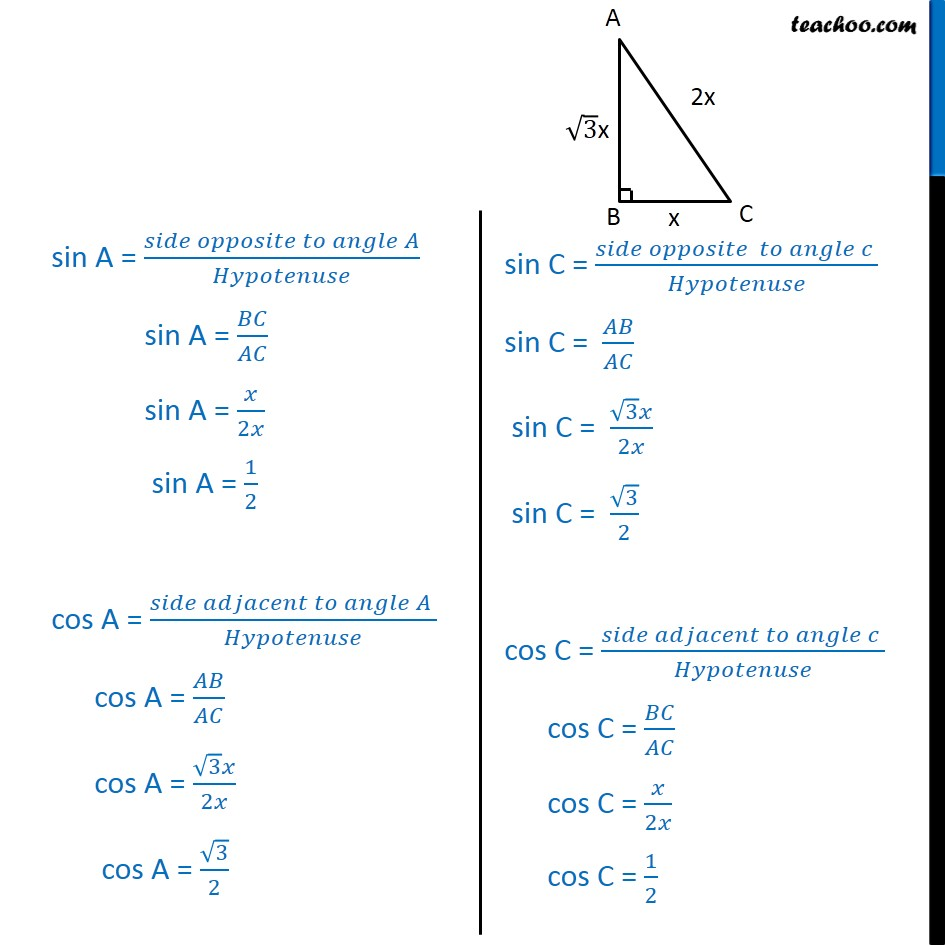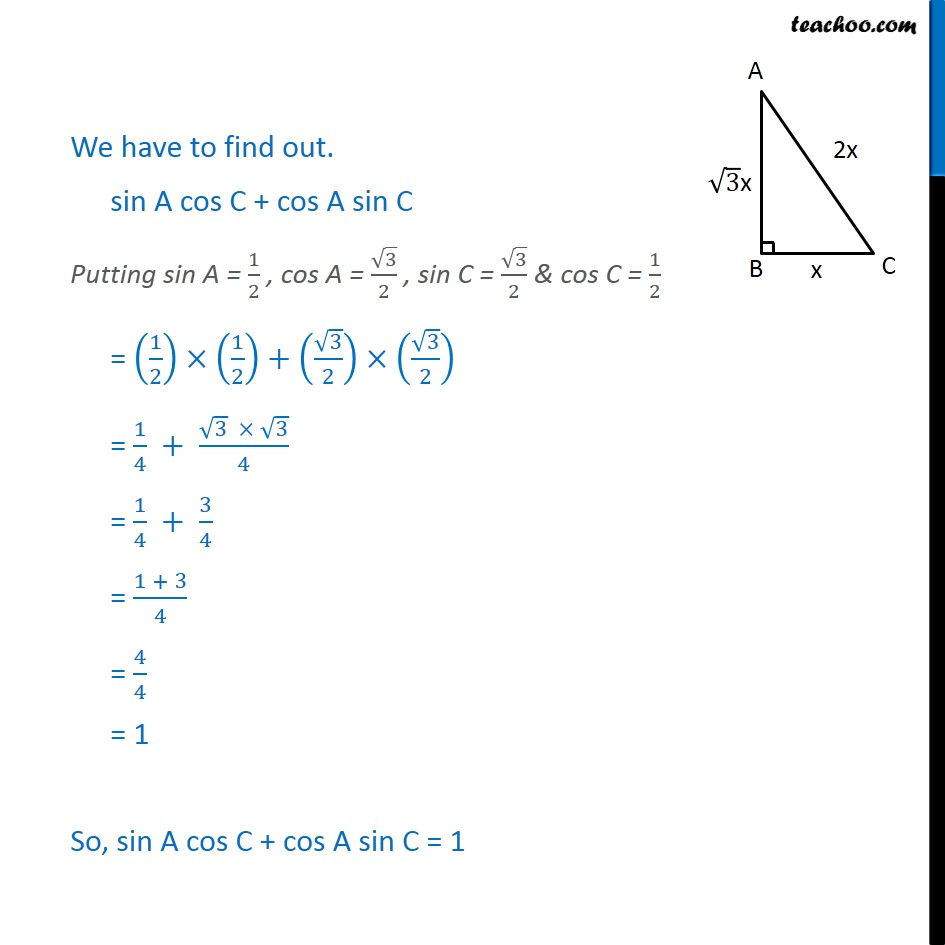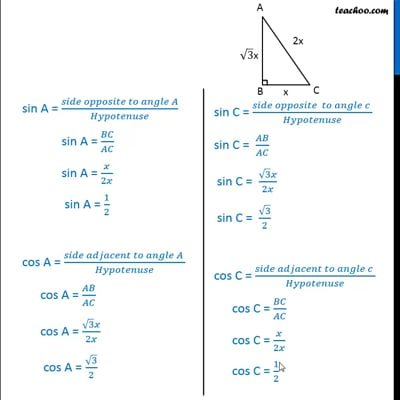This video is only available for Teachoo black users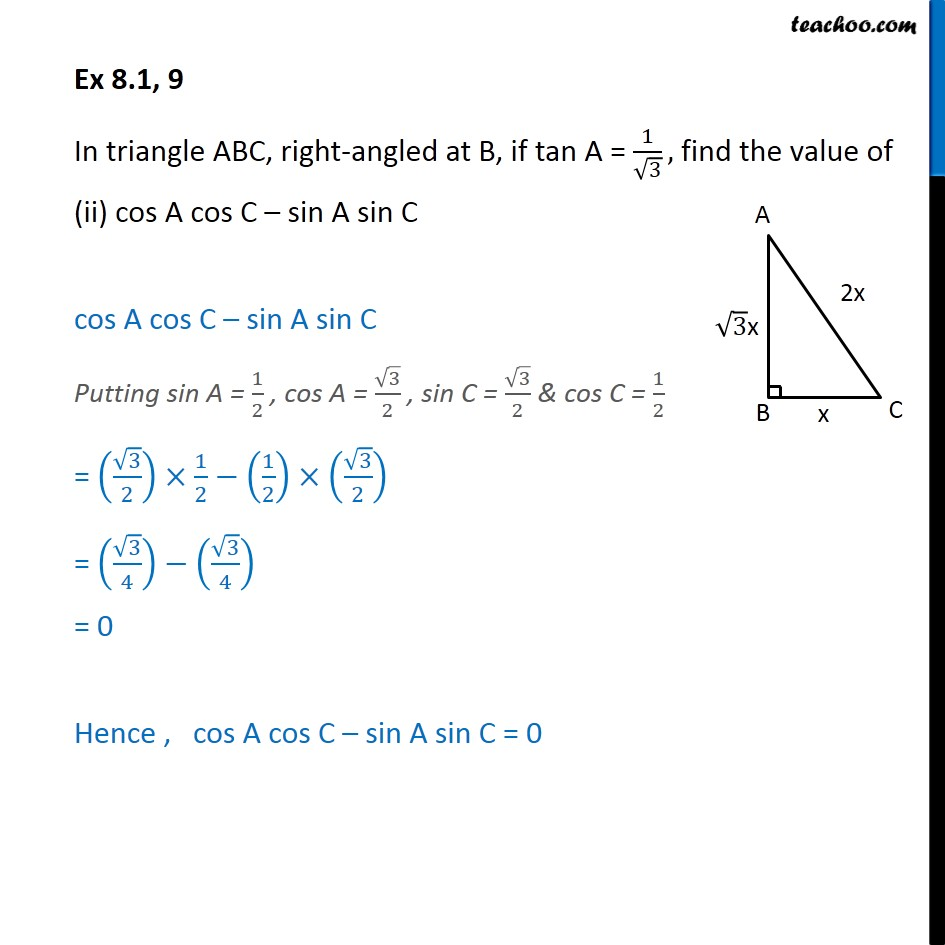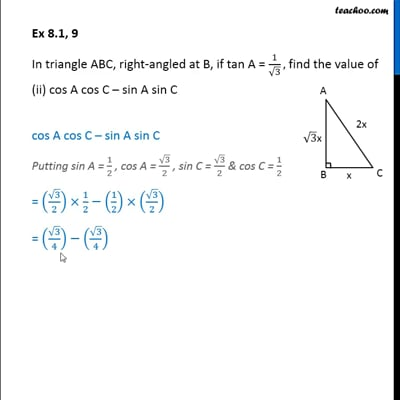This video is only available for Teachoo black users

### Transcript

Ex 8.1, 9 In triangle ABC, right-angled at B, if tan A = 1/√3, find the value of sin A cos C + cos A sin C tan A = 1/√3 (𝑠𝑖𝑑𝑒 𝑜𝑝𝑝𝑜𝑠𝑖𝑡𝑒 𝑡𝑜 𝐴)/(𝑠𝑖𝑑𝑒 𝑎𝑑𝑗𝑎𝑐𝑒𝑛𝑡 𝑡𝑜 𝐴) = 1/√3 𝐵𝐶/𝐴𝐵 = 1/√3 Let BC = x & AB = √3 x We find AC using Pythagoras theorem Using Pythagoras theorem (Hypotenuse)2 = (Height)2 + (Base)2 AC2 = AB2 + BC2 AC2 = (√3 𝑥)^2+(𝑥)2 AC2 = 3x2 + x2 AC2 = 4x2 AC = √4𝑥2 AC = 2x We need to find sin A , cos A , sin C & cos C sin A = (𝑠𝑖𝑑𝑒 𝑜𝑝𝑝𝑜𝑠𝑖𝑡𝑒 𝑡𝑜 𝑎𝑛𝑔𝑙𝑒 𝐴)/𝐻𝑦𝑝𝑜𝑡𝑒𝑛𝑢𝑠𝑒 sin A = 𝐵𝐶/𝐴𝐶 sin A = 𝑥/2𝑥 sin A = 1/2 cos A = (𝑠𝑖𝑑𝑒 𝑎𝑑𝑗𝑎𝑐𝑒𝑛𝑡 𝑡𝑜 𝑎𝑛𝑔𝑙𝑒 𝐴 )/𝐻𝑦𝑝𝑜𝑡𝑒𝑛𝑢𝑠𝑒 cos A = 𝐴𝐵/𝐴𝐶 cos A = (√3 𝑥)/2𝑥 cos A = √3/2 We have to find out. sin A cos C + cos A sin C Putting sin A = 1/2 , cos A = √3/2 , sin C = √3/2 & cos C = 1/2 = (1/2)×(1/2)+(√3/2)×(√3/2) = 1/4 + (√3 × √3)/4 = 1/4 + 3/4 = (1 + 3)/4 = 4/4 = 1 So, sin A cos C + cos A sin C = 1 Ex 8.1, 9 In triangle ABC, right-angled at B, if tan A = 1/√3, find the value of (ii) cos A cos C – sin A sin C cos A cos C – sin A sin C Putting sin A = 1/2 , cos A = √3/2 , sin C = √3/2 & cos C = 1/2 = (√3/2)×1/2−(1/2)×(√3/2) = (√3/4)−(√3/4) = 0 Hence , cos A cos C – sin A sin C = 0